# Percentages + unit conversion - examples

1. RiverCalculate how many promiles river Dunaj average falls, if on section long 957 km flowing water from 1454 m AMSL to 101 m AMSL.
2. ServerCalculate how many average minutes a year is the web server is unavailable, the availability is 99.99%.
3. TanksFire tank has cuboid shape with a rectangular floor measuring 13.7 m × 9.8 m. Water depth is 2.4 m. Water was pumped from the tank into barrels with a capacity of 2.7 hl. How many barrels were used, if the water level in the tank fallen 5 cm? Wr
4. Climb in percentageThe height difference between points A and B is 475 m. Calculate the percentage of route climbing if the horizontal distance places A, B is 7.4 km.
5. Peroxide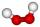How much distilled water (in liters) must pharmacists pour into 300 ml of 23.6% solution of hydrogen peroxide to get 2.7% solution to gargle?
6. Solidarity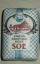Imagine a word solidarity means that salt donation to the needy, who have neither the salt. If we take word solidarity has word base salt + gift (only in Slovakian language). Calculate how many kilos of salt sympathetic citizen "gives" government a year i
7. Built-up areaJohn build up area 5 x 7 = 35 m2 with building with a wall thickness 30 cm. How many centimeters would have to subtract from thickness of the walls that built-up area fell by 9%?
8. Glass mosaicHow many dm2 glass is nessesary to produc 97 slides of a regular 6-gon, whose side has length 21 cm? Assume that cutting glass waste is 10%.
9. IcerinkRectangular rink with dimensions of 68.7 m and 561 dm must be covered with a layer of ice 4.2 cm thick. How many liters of water is necessary for the formation of ice when the volume of ice is 9.7% greater than the volume of water.
10. VintnerHow high can vintner fill keg with crushed red grapes if these grapes occupy a volume of 20 percent? Keg is cylindrical with a diameter of the base 1 m and a volume 9.42 hl. Start from the premise that says that fermentation will fill the keg (the number.
11. Temperature calculus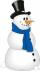Calculate percentage of temperature increase if changed from -9°C to 22°C?
12. Bronze, tin and copper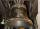Bronze is an alloy of tin and copper. An alloy of 10% tin and 90% copper is Gunmetal. If it contains 20% tin and 80% copper, it is bell metal. How many tons of molten bell metal and how many tons of copper is needed to make 100 tons of Gunmetal?
13. Work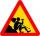Workers works 5 days a week and has 21 days of vacation a year. Calculate how many days is out of work and express it as a percentage. Consider that a year has 52 weeks.
14. BricksOpenings in perforated bricks occupy 10% and brick has dimensions 30 cm, 15 cm and 7.5 cm. Calculate a) the weight of a perforated bricks, if you know that the density of the full brick material is p = 1800 kg/m3 (1.8 kg/dm3) b) the number of perforated.
15. Water tank 2Water tank cuboid is 12 meters long and 6.5 meters wide and 1.2 meters high. How many hectoliters are in the tank when it is filled to 81%?
16. HallRectangular hall will have pave by square tiles with a side length 15 cm. The hall has length 18 meters and width 3 m. How many tiles need to buy if 2 percent of the amount is disrupted during the work?
17. Wood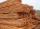Wood contains 12% water. 1 m3 of wood weighs 650 kg. How many liters of water it contains?
18. UV radiation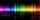The glass of 1 mm thickness absorb 4% of UV radiation passing through. How many percent of UV rays absorb glass with a thickness 1.1 cm, made from 1 mm thick those glasses?
19. Metal box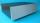How much metal we need for the production of box with dimensions 5 dm, 30 cm and a height of 1 m? Add 12% of the waste and folds.
20. Sugar cubesThe glass has 600 ml of tea, which represents 80% of the volume of the glass. If you put twenty regular sugar cubes of 2 cm in the tea, how many ml of tea are poured?

Do you have an interesting mathematical example that you can't solve it? Enter it, and we can try to solve it.

To this e-mail address, we will reply solution; solved examples are also published here. Please enter e-mail correctly and check whether you don't have a full mailbox.

Our percentage calculator will help you quickly calculate various typical tasks with percentages.